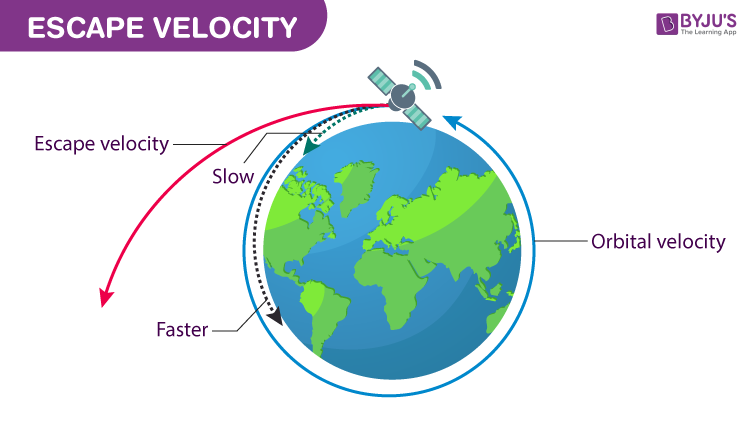Checkout JEE MAINS 2022 Question Paper Analysis : Checkout JEE MAINS 2022 Question Paper Analysis :

# Escape Speed

Escape speed is the minimum speed required to escape a planet’s gravitational pull.

A spacecraft leaving the earth’s surface should be going at a speed of about 11 kilometres (7 miles) per second to enter the outer orbit. Here, in this article let us dig deeper into the concept of escape speed.

## What is Escape Speed?

Escape speed is the minimum speed with which a mass should be projected from the Earth’s surface in order to escape Earth’s gravitation field. Escape speed, also known as escape velocity is defined as:

The minimum speed that is required for an object to free itself from the gravitational force exerted by a massive object.For example, if we consider earth as a massive body. The escape velocity is the minimum velocity that an object should acquire to overcome the gravitational field of earth and fly to infinity without ever falling back.

It purely depends on the distance of the object from the massive body and the mass of the massive body. More the mass it will be higher, similarly, the closer distance, higher will be the escape velocity.

For any massive bodies such as planets, stars which are spherically symmetric in nature, the escape speed for any given distance is mathematically expressed as:

 $$\begin{array}{l}v_{e}=\sqrt{\frac{2GM}{r}}\end{array}$$

Where,

• ve is the escape speed
• G is the universal gravitational constant (G≅6.67×10-11 m3kg-1s-2)
• M is the mass of the massive body(the body from which the object is to be escaped from)
• r is the distance from the centre of the massive body to the object

Here we can notice that the above-mentioned relation is independent of the mass of the object which will be escaping from the massive body.

You may also want to check out these topics given below!

## Derivation of Escape Speed

In general escape, speed is achieved when the object moves with a velocity at which the arithmetic sum of the object’s gravitational potential energy and its Kinetic energy equates to zero. That is, the object should possess greater kinetic energy than the gravitational potential energy to escape to infinity.

• The simplest way of deriving the formula is by using the concept of conservation of energy. Let us assume that the object is trying to escape from a planet (which is uniform circular in nature) by moving away from it.
• The prime force acting on such an object will be the planet’s gravity. As we know, Kinetic energy(K) and the Gravitational Potential Energy(Ug) are the only two kinds of energies associated here.

By the principle of conservation of energy, we can write:

$$\begin{array}{l}\left ( K+U_{g} \right )_{i}=\left ( K+U_{g} \right )_{f}\end{array}$$

Where,

• $$\begin{array}{l}K=\frac{1}{2}mv^{2}\end{array}$$
• $$\begin{array}{l}U=\frac{GMm}{r}\end{array}$$

Here Ugf is zero as the distance is infinity and Kf will also be zero as final velocity will be zero. Thus, we get:

$$\begin{array}{l}\frac{1}{2}mv_{e}^{2}-\frac{GMm}{r}=0+0\end{array}$$
$$\begin{array}{l}\frac{1}{2}mv_{e}^{2}=\frac{GMm}{r}\end{array}$$
$$\begin{array}{l}\Rightarrow v_{e}=\sqrt{\frac{2GM}{r}}\end{array}$$

The minimum velocity required to escape from the gravitational influence of massive body is given by:

$$\begin{array}{l}v_{e}=\sqrt{2gr}\end{array}$$

Where,

$$\begin{array}{l}g=\frac{GM}{r^{2}}\end{array}$$

The escape speed of the earth at the surface is approximately 11.186 km/s. That means “an object should have a minimum of 11.186 km/s initial velocity to escape from earth’s gravity and fly to infinite space.”

Ideally, If you can jump with initial velocity 11.186 km/s you can tour outer space! Isn’t it interesting? For more such brain-twisting concepts do follow the links given below.

## Unit Of Escape Speed

Unit of escape speed or the escape velocity is metre per seconds (m.s-1) which is also the SI unit of escape speed.

### Dimensional Formula:

 Dimensional formula of universal gravitational constant = M-1L3T-2 Dimensional formula of the mass of the earth = M1L0T0 Dimensional formula of distance to the centre of the earth = M0L1T0 Therefore, the dimensional formula of escape speed after substituting in the equation is = M0L1T-1

1) Is it better to launch a ship into the orbit from near or away from the equator?

Ans: It is better to launch a ship from the equator because the radius is greater at the equator than at the poles. This lowers the escape velocity.

2) Compute the escape velocity for the indicated planet. Use G = 6.67 x 10-11 N-m2/kg2

a) Mars: Mass 6.46 x 1023 kg; Radius 3.39 x 106 m

Solution:

The formula to find the escape speed is as follows:

$$\begin{array}{l}v_{e}=\sqrt{\frac{2GM}{r}}\end{array}$$

Substituting the values in the equation, we get

$$\begin{array}{l}v_{e}=\sqrt{\frac{2(6.67\times 10^{-11})(6.46\times 10^{23})}{3.39\times 10^6}}\end{array}$$
$$\begin{array}{l}\sqrt{25420766}\end{array}$$
$$\begin{array}{l}\approx 5.04\times 10^3\end{array}$$

The escape speed for earth is approximately equal to 5.04 x 103 m/s.

Test your knowledge on Escape speed